Checkout NEET 2022 Question Paper Analysis : Checkout NEET 2022 Question Paper Analysis :

# Laws of motion MCQs for NEET

Newton’s laws of motion are the fundamental laws that are used for describing the motion of the body. There are three laws of motion and they are as follows:

• An object continues to be in the state of uniform motion or in the state of rest unless an external force acts on it. (Also known as the law of inertia)
• Force is a product of mass and acceleration.
• For every action, there is an equal and opposite reaction. (Also known as conservation of momentum)

1. A particle of mass m is given an impulse such that the velocity of the particle changes from v1 to v2. The impulse is equal to

(a) m(v2 + v1)

(b) m (v2 – v1)

(c) m (|v2|-|v1|)

(d)

$$\begin{array}{l}\frac{1}{2}(v_{2}^{2}-v_{1}^{2})\end{array}$$

Answer: (b) m (v2 – v1)

2.What is the time taken by the body to slide down an inclined plane if the length of the inclined plane is L, a as the retardation, and Ө is the angle of inclination.

(a)

$$\begin{array}{l}\sqrt{\frac{2L}{g\;sin\theta }}\end{array}$$

(b)

$$\begin{array}{l}\sqrt{\frac{2L}{a\;sin\theta }}\end{array}$$

(c)

$$\begin{array}{l}\sqrt{\frac{2L}{(g+a)\;sin\theta }}\end{array}$$

(d)

$$\begin{array}{l}\sqrt{\frac{2L}{(g-a)\;sin\theta }}\end{array}$$

$$\begin{array}{l}\sqrt{\frac{2L}{(g+a)\;sin\theta }}\end{array}$$

3. Choose the correct statement

(a) A body can be accelerated by frictional force

(b) There can be zero friction

(c) Kinetic friction is greater than rolling friction

(d) Frictional force and area of contact between the two surfaces are proportional

Answer: (c) Kinetic friction is greater than rolling friction

4. Consider a car that overturns while taking a turn. Select the option that is experienced by the wheels of the car.

(a) First, both inner, as well as the outer wheel, leave the ground

(b) First, the inner wheel leaves the ground

(c) The inner wheel experiences no change

(d) None of the above

Answer: (b) First, the inner wheel leaves the ground

5. Let two bodies be with masses 2kg and 5kg respectively. Let these bodies be at rest with the same force acting on them. Calculate the ratio of times that is required by both the bodies to reach the final velocity.

(a) 2:5

(b) 25:4

(c) 5:3

(d) 4:25

6. What is the force acting on the particle if the motion of the particle is given as

$$\begin{array}{l}y=ut+\frac{1}{2}gt^{2}\end{array}$$

(a) F = ma

(b) F = 0

(c) F = mg

(d) F ≠ 0

7. Consider two bodies of masses A and B such that these two bodies are connected by using a massless spring. Also, force F is acting on the body B. What will be the acceleration of B if A has an acceleration of a?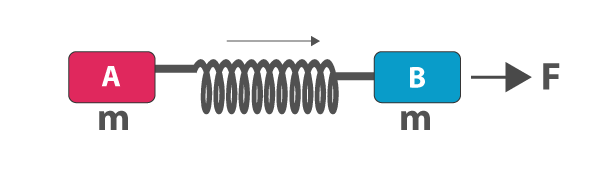(a) a

(b) F – T

(c)

$$\begin{array}{l}(\frac{F}{m}-a)\end{array}$$

(d)

$$\begin{array}{l}(a-\frac{F}{m})\end{array}$$

$$\begin{array}{l}(\frac{F}{m}-a)\end{array}$$

8. Let A, B, and C be the three blocks of mass 2kg each. These blocks are hanging over a pulley with the help of a string. What is the tension produced by the string connecting B and C?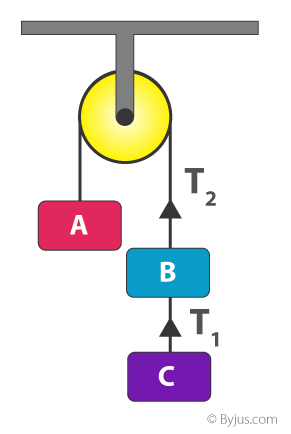(a) 3.3N

(b) 0

(c) 13.1N

(d) 19.6N

9. Consider three masses of 1kg, 3kg and 6kg which are connected to each other and are placed horizontally on the table. Calculate the acceleration of this system. Consider g = 10m/s.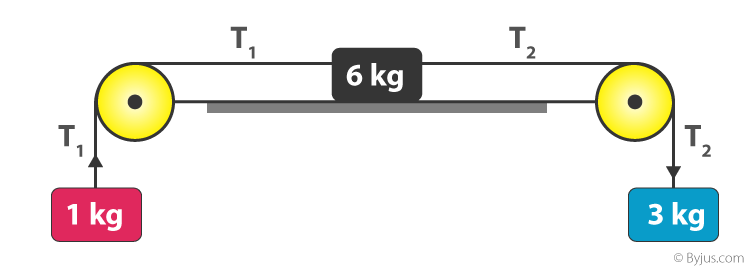(a) 3

(b) 0

(c) 1

(d) 2

10. From the following diagram, select the correct diagram when a vessel containing water is given a constant acceleration towards the right along the straight line.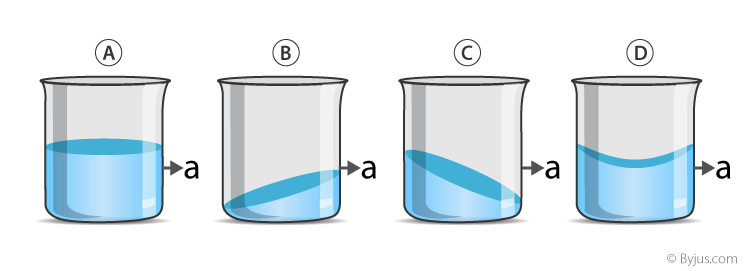Answer: (c)

11. Consider a man standing on the spring balance such that the reading on the spring balance is 60kg. If the man jumps out of the spring balance what will be the final reading of the spring balance?(a) Remains unchanged

(b) Increases

(c) Decreases

(d) First increases then decrease to zero

Answer: (d) First increases then decreases to zero

12.Which of the following should be constant for a body to have a constant momentum?

(a) Acceleration

(b) Force

(c) Velocity

(d) All of the above

Answer: (d) All of the above

### Recommended Video:

Simple Harmonic Motion Class 11 Physics One Shot – Concepts & Problems | NEET 2022 Physics Exam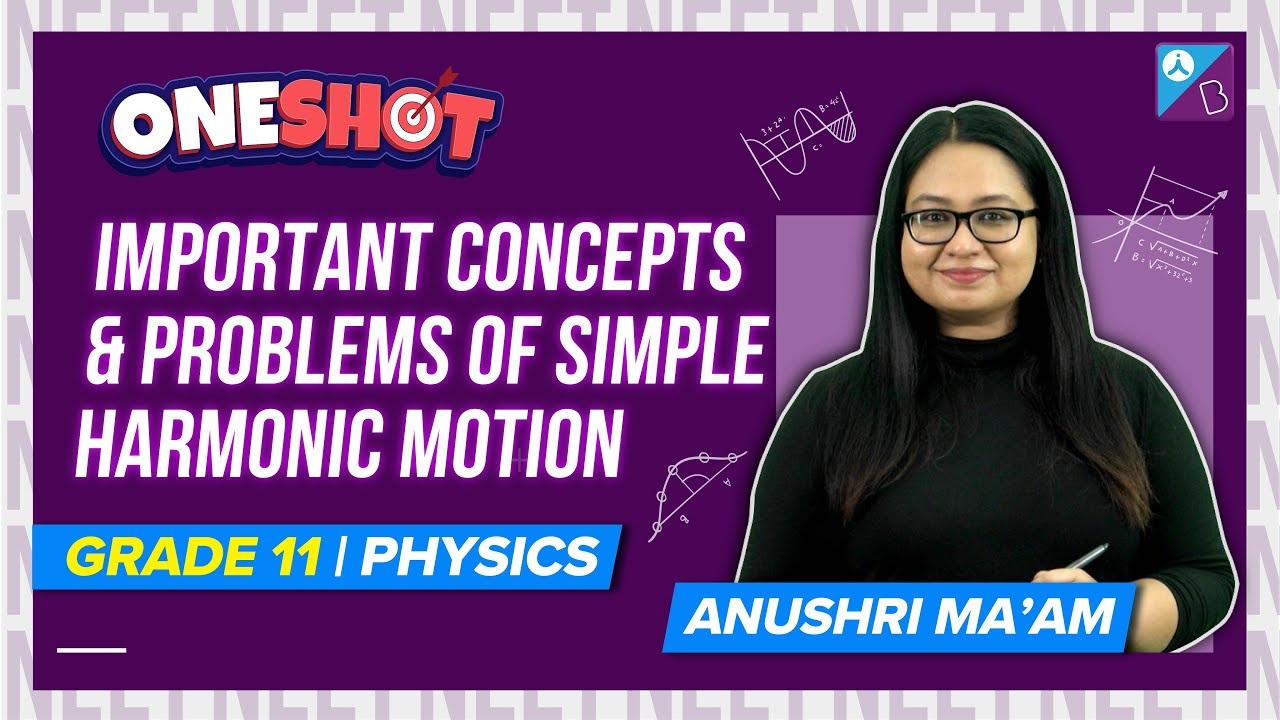More NEET Physics MCQs:

#### 1 Comment

1. govind gupta

I like it